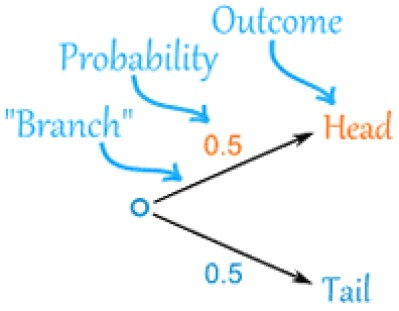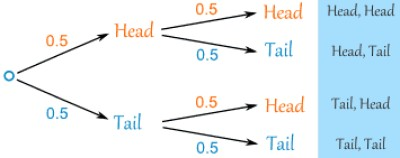List of public pages created with Protopage

## Plain sticky notes

i don't even know ¯\_(ツ)_/¯

## Photos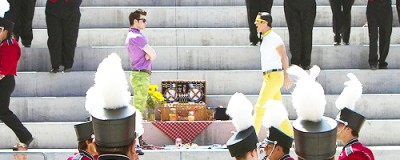# Estimation

## Plain sticky notes

Estimation
Generally, you should round each number involved to one significant figure and then it's easy to estimate by using the single digits and moving the point around.
It is important to remember that...
..most measurement is approximate! - If you say your garden is 8 metres long you are rounding to the nearest metre and it could be anything from 7.5 to 8.5 metres long!
Lower & Upper Bounds
The real value can be as much as half the rounded unit above or below the value given. So, if you are given 5.4cm the upper bound is 5.45cm and the lower bound is 5.35cm. For 6.0kg you need to go 0.05kg either way so the upper bound is 6.05kg and the lower bound is 5.95kg.
Video example ↓

## Photos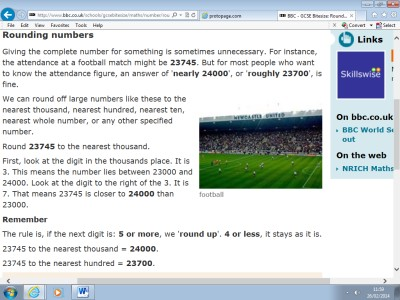# Area of Triangles

## Plain sticky notes

Area of Triangles
Heron's formula
The height is called the perpendicular height because it is at a right-angle to the base. Below is an example of the formula being used to find the area of a triangle.

## Photos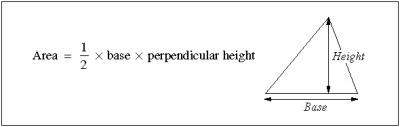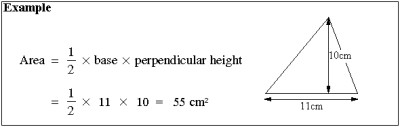# Vectors

## Plain sticky notes

Vectors
A vector is a way to represent a movement between two places.
↓ Video examples
We visualise vectors by using straight lines with arrows on.

# Notes

## Plain sticky notes

### Sticky note

Click this text to change it...

# Graphs

## Plain sticky notes

Graphs
Cubic ↓

### Sticky note

Straight Line ↓
An example of a straight line graph is: y=x+7 X -2 -1 0 1 2 3 4 5 6 7 8 9 10 Y 5 6 7 8 9 10 11 12 13 14 15 16 17 This is shown, plotted, below.

## Photos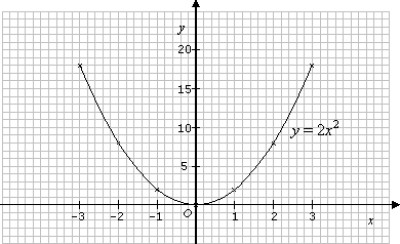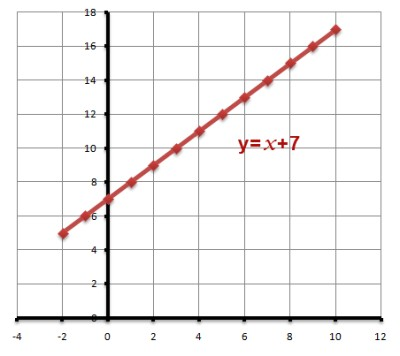# Histograms

## Plain sticky notes

Histograms
Histograms can have bars of different widths.
Videos ↓
Formulas →
FD = F ÷ CW CW = F ÷ FD F = FD x CW

## Photos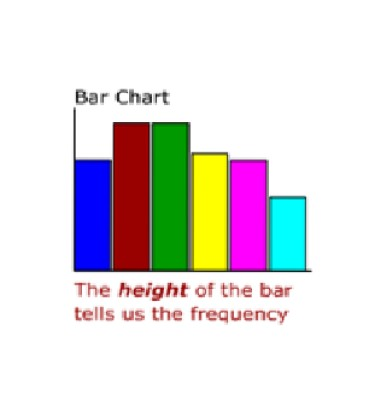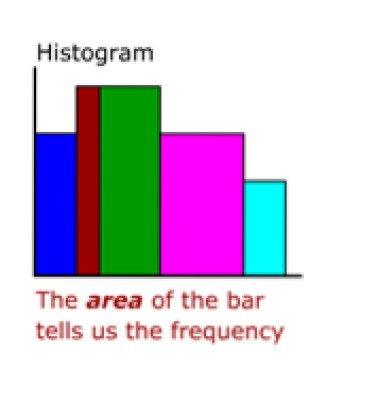Circle Theorems

# Simultaneous Equations

## Plain sticky notes

Simultaneous Equations

# Bookmarks

## Plain sticky notes

### Sticky note

Click 'edit' on the Bookmarks widget to add all of your favorite sites to your page for quick access

# Sectors & Arcs

## Plain sticky notes

Sectors & Arcs
Sectors
Arcs
Circumference = πd or 2πr
circumference = 10π cm area = 25 π cm2 and the angle 72° is 1/5 of 360° Arc length = 1/5 of 10 π = 2 π = 6.283cm (3 d.p.) Sector area = 1/5 of 25 π = 5 π = 15.708cm2 (3 d.p.)

## Photos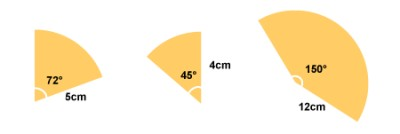# Probability Trees

## Plain sticky notes

Probability Trees
This shows the toss of a coin..
The two possible outcomes (Heads and Tails) are AT THE END of the branches The probability of each branch is written ON the branch
This can be extended to two tosses of a coin..
You multiply probabilities along the branches Head, Head = 0.5 x 0.5 = 0.25 Head, Tails = 0.5 x 0.5 = 0.25 Tails, Head = 0.5 x 0.5 = 0.25 Tails, Tails = 0.5 x 0.5 = 0.25
The probability of getting at least one Head from two tosses is: 0.25+0.25+0.25 = 0.75

## Photos